Transcripted Summary

Hey, hello everyone. Welcome back.

In this video, we will see about unique tests features Mocha provides us with.

There are 3 types of test features available. First, exclusive. Second, inclusive. And third, pending.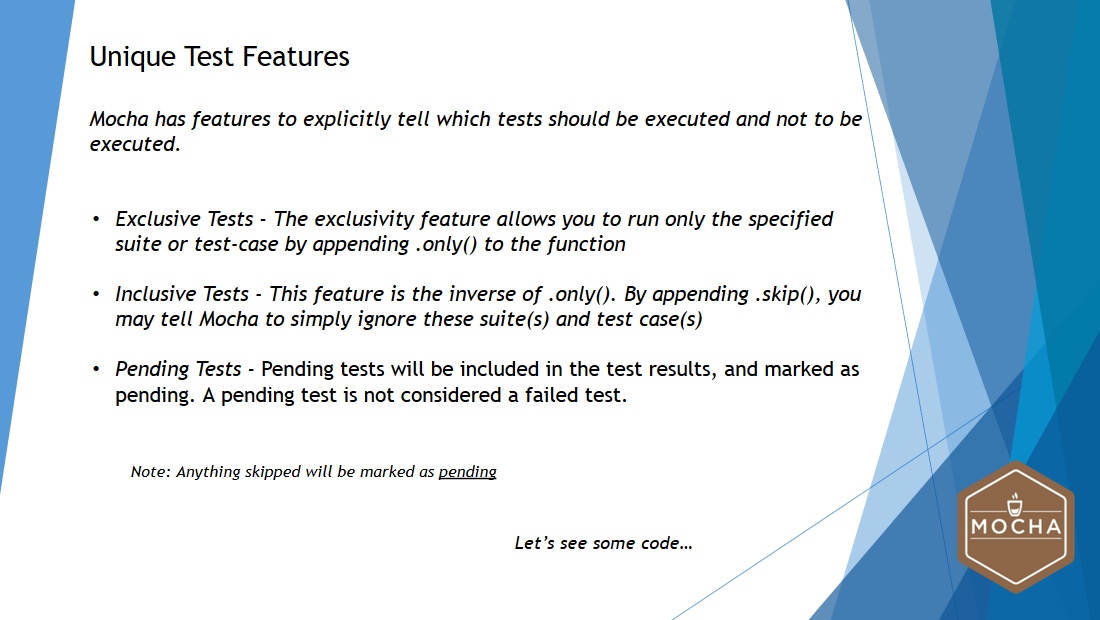Firstly, exclusive test feature allows us to execute only the specified test suite or a test case.

This can be achieved by adding `.only()` function, and it can be added to both `describe()` and `it()` functions.

Inclusive test is an inverse of the `.only()` function.

This can be achieved by adding `.skip()`. This will tell Mocha to simply ignore these tests suites or test cases. This is applicable for both pre-bundled functions, `describe()` and `it()`.

Pending tests are the ones that someone should write eventually.

Pending tests will be included in the test results and marked as pending. A pending test is not considered as failed a test.

Now let's see all these three unique test features in the code.

### # Using Mocha’s Unique Test Features

I have taken the “Mathematical Operations - Test Suite”, which we drafted earlier.

So, in this test suite, we have fo4ur different test cases — all 4 different test cases test 4 different operations like addition, subtraction, multiplication, and division.

Before we get into the unique test features concept, let's run this and see whether all the test cases are getting executed.

I'm launching my terminal and typing the command:

``````npm test
``````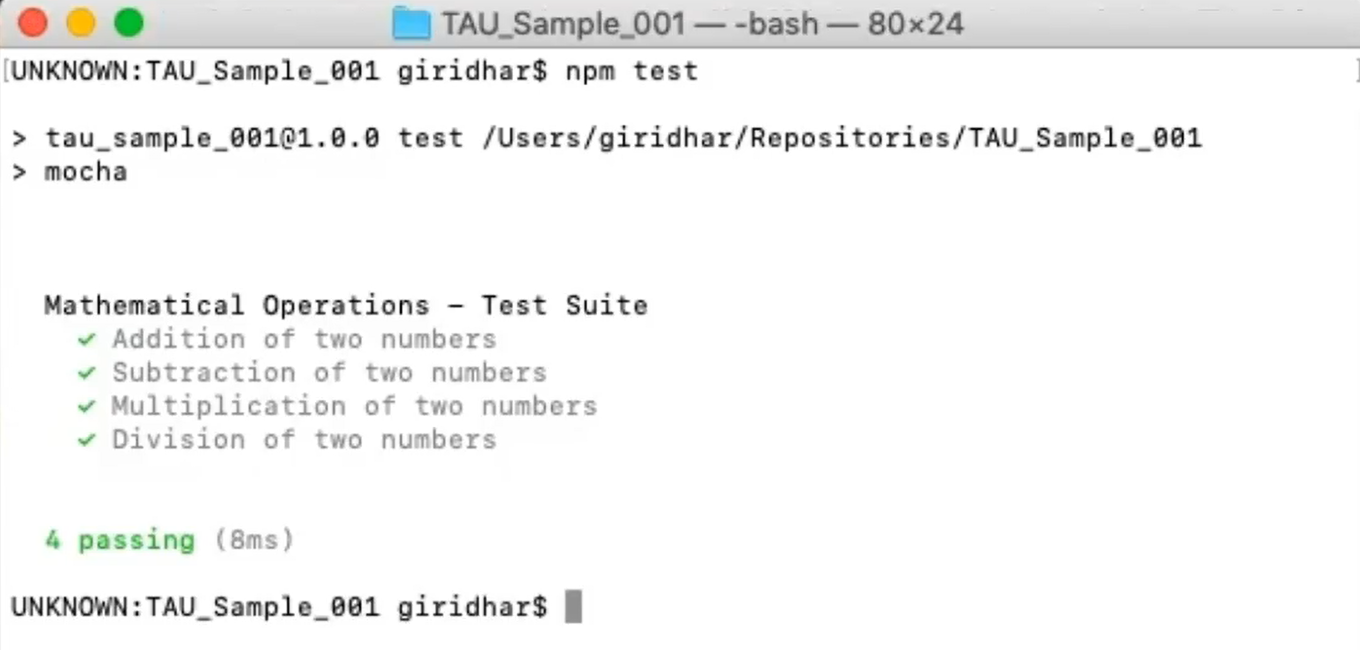So, I'm able to see that all 4 test cases are getting executed now.

Now let's see the first feature — exclusive test.

Like we saw earlier, in order to execute only test case, we need to add `.only()` function to that particular test case.

So, in my scenario, I'm going to execute only the addition test case.

To do that, I'm just typing — `it.only` — this means that if I execute the test case via terminal, only the addition test case will be executed, and the rest of the three test cases will not be executed.

``````var assert = require('assert');

describe('Mathematical Operations - Test Suite', function(){
var a = 10;
var b = 10;

var c = a+b;
assert.equal(c,20);
});
``````

Let me rerun the test now.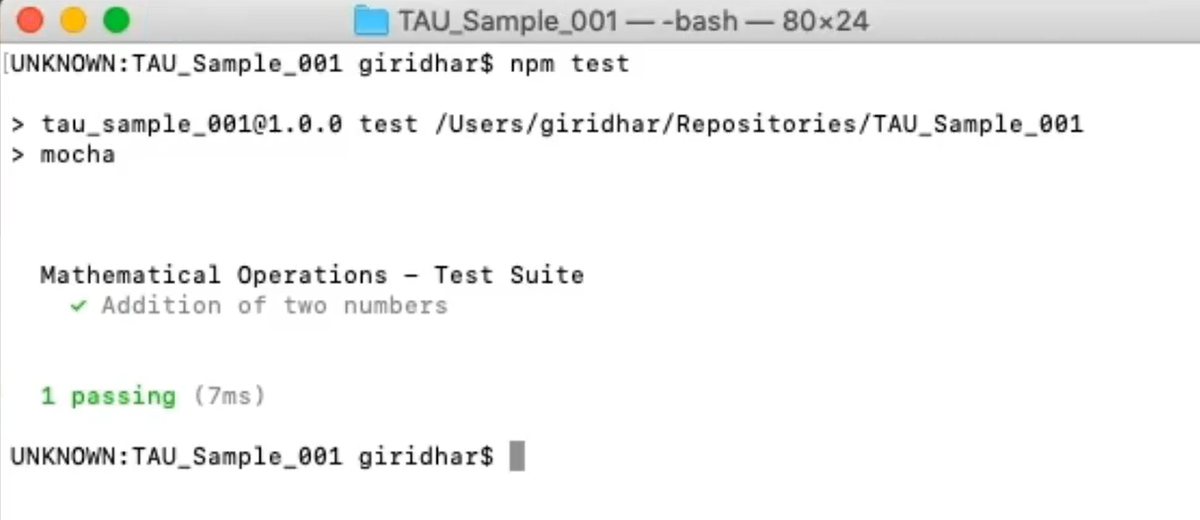So, if you see here, only the addition of 2 numbers test case got executed where we have given the `.only()` function.

Now let's try to add the `.only()` function to the other test case, like subtraction.

So here, I'm going to give again `.only()`:

``````it.only('Subtraction of two numbers', function(done){

}
``````

And to execute it, I'm typing:

``````npm test
``````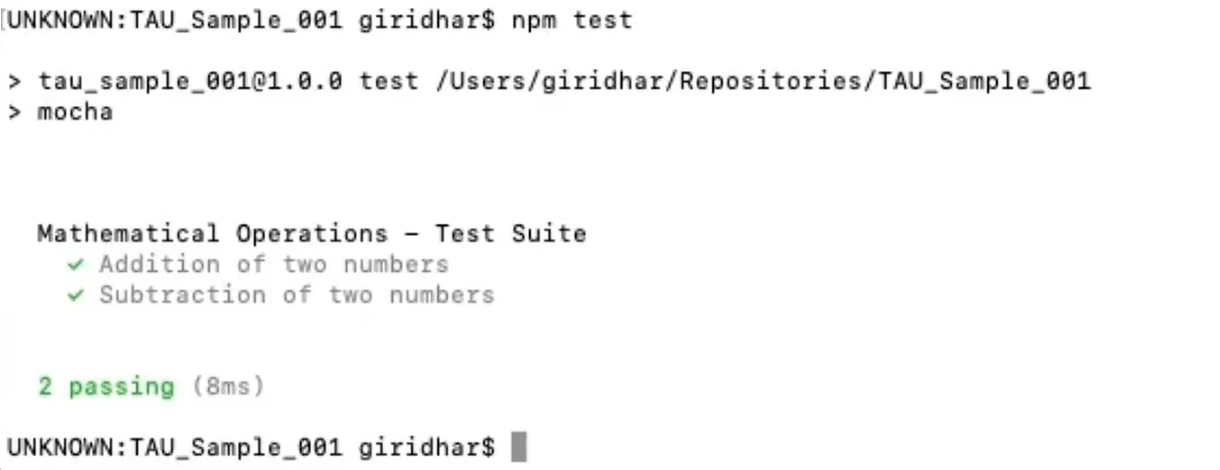If you see here, the 2 functions or the 2 test cases that we want to execute have code executed here.

This is by adding `.only()` function to the test case.

Now let's see the second type of test feature that we have seen, the inclusive test.

We have seen that in order to use inclusive test, we need to add the function `.skip()`.

So here, if I want to execute only the multiplication test case [as well as the subtraction and division tests], all I have to do is just put `.skip()` [on the addition test case].

``````var assert = require('assert');

describe('Mathematical Operations - Test Suite', function(){
var a = 10;
var b = 10;

var c = a+b;
assert.equal(c,20);
});

``````

Now that we have added `.skip()`, let's try to execute this.

To execute this, I'm opening my terminal again. Once I launched my terminal, I am typing the command again,

``````npm test
``````

And when I hit Enter, we will be able to see that the addition of 2 numbers test case, since we have given it as `.skip()`skip, it got skipped and automatically it has become pending.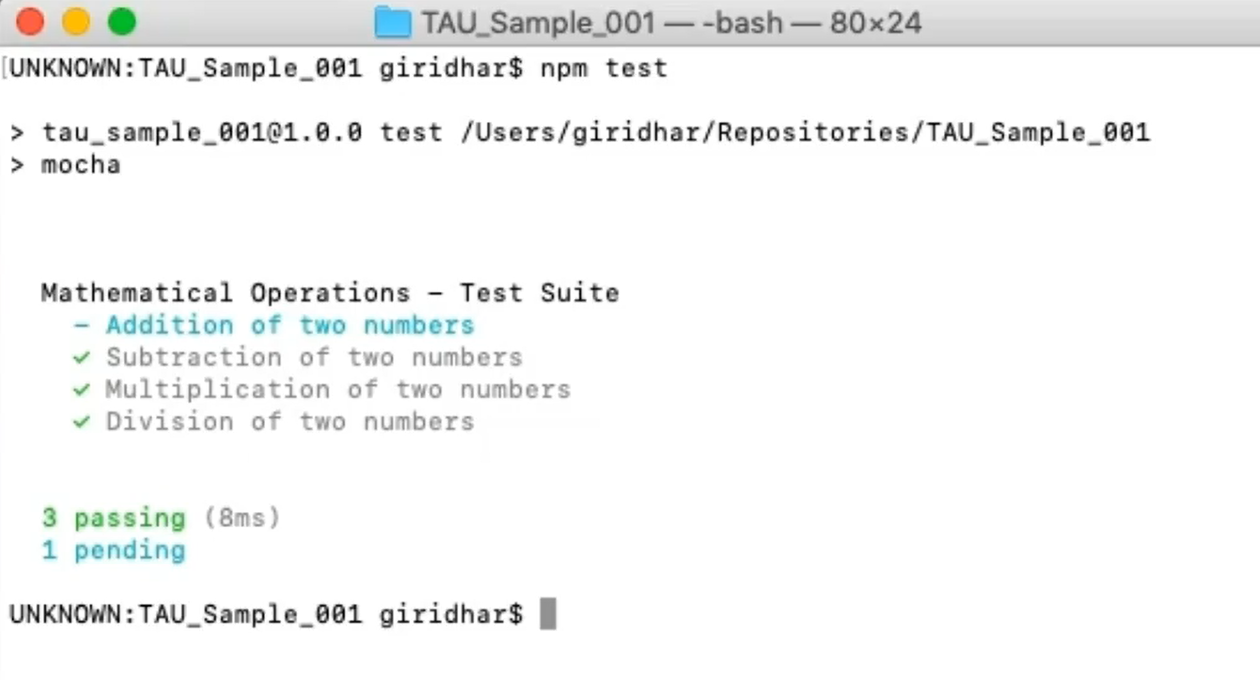Whereas the other test cases where we haven't given any test features, it got executed successfully.

Now that we have seen inclusive and exclusive tests, we will now see what is pending test.

So basically, we have seen already that a pending test will not be having a callback `function`.

Hence, if we execute a test without a callback `function`, that particular test will become pending.

Let's see that in action.

``````   // Pending Test
it('This is a test for Pending Test Feature');
``````

So, I have written a fifth test case with the `it()` function without a callback `function` here.

And if I execute this particular test suite, automatically I will be having 4 tests executed and 1 test in pending state.

Let's see that now — I'm opening the terminal and executing the test.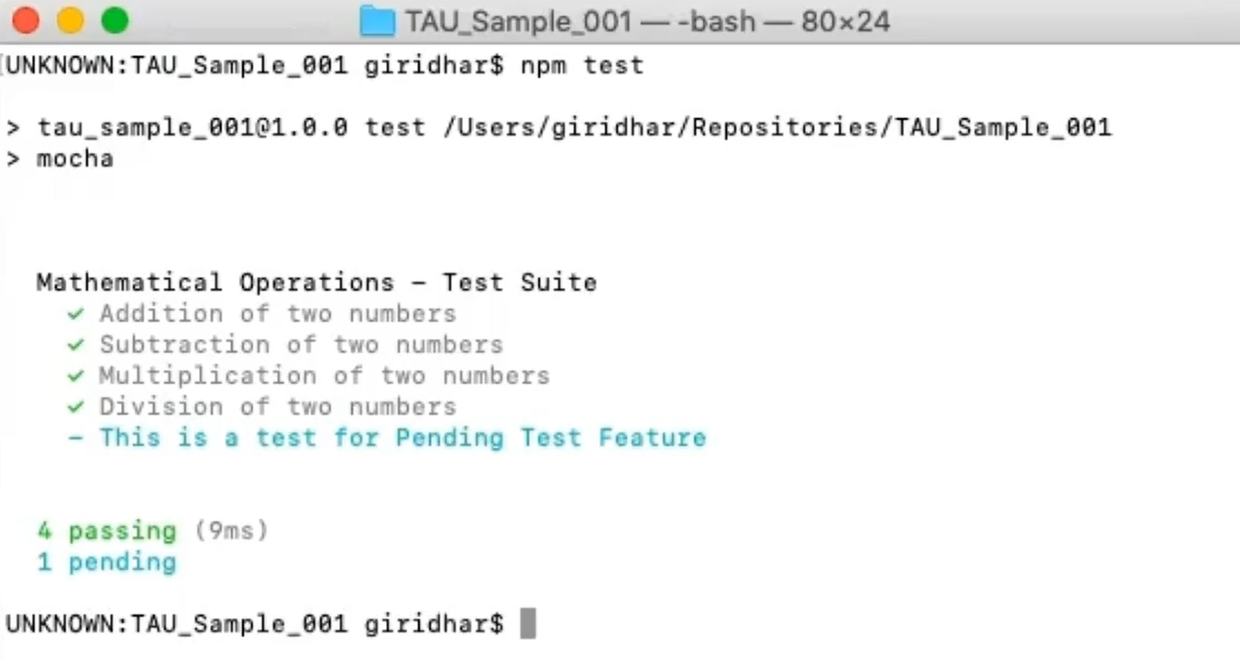Here you will be able to see that the previous test cases got executed successfully and the 1 which we have created without a callback function has become a pending test.